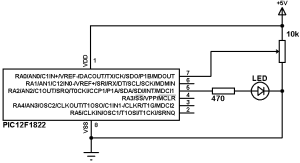# PIC12F1822 ADC and PWM modules

This topic gives a short descriptions about PIC12F1822 microcontroller ADC and PWM module and how to use them using CCS PIC C compiler. For more details go to PIC12F1822 datasheet.

PIC12F1822 microcontroller has a 10-bit ADC (Analog-to Digital Converter) module. 4 Pins can be used as analog inputs which are: RA0 (AN0), RA1 (AN1) RA2 (AN2) a,d RA4 (AN3).
The ADC module is used to read analog data comes from analog devices like analog potentiometer and analog temperature sensors ……
The following CCS C line is used to configure PIC12F1822 ADC module:
Where mode is the source of the conversion clock (ADC clock source). There
are seven possible clock options:
• FOSC/2
• FOSC/4
• FOSC/8
• FOSC/16
• FOSC/32
• FOSC/64
• FRC (dedicated internal oscillator)
CCS PIC C compiler uses the following constants for the previous parameters (used with setup_adc():
And to turn off the ADC use the following constant:

Example:
The following command is used to configure a digital input pin as analog:
The following constants are used in the previous function:
sAN0                                 // Configure AN0 as analog
sAN1                                 // Configure AN0 as analog
sAN2                                 // Configure AN0 as analog
sAN3                             // Configure AN0 as analog
NO_ANALOGS                      // All pins are configured as digital
ALL_ANALOGS                     // All pins are configured as analog

Examples:
setup_adc_ports(sAN1);                                   // Configure AN1 as analog
setup_adc_ports(sAN0 | sAN3);                               // Configure AN0 and AN3 as analog
To read analog data the analog channel must be selected first using this line:
Where channel can be: 0, 1, 2 or 3.

Example:
set_adc_channel(2);               // Select analog channel 2
And to read data use the following line:

PIC12F1822 PWM module:
PIC12F1822 microcontroller has 1 ECCP module (Enhanced Capture/Compare/PWM) which allows us to generate Pulse-Width Modulation (PWM) signals.
The PWM period is specified by the PR2 register of Timer2. The PWM period can be calculated using the following equation:
PWM period = [(PR2) + 1] • 4 • Tosc • (TMR2 Prescale Value)
And the PWM frequency is defined as 1/[PWM period].
Tosc = 1/(MCU_frequency)
TMR2 Prescale Value can be 1, 4, 16 or 64.
For example for PR2 = 255 , microcontroller frequency = 8MHz and Prescale = 16 we get a PWM frequency of 488Hz.
The CCS Timer2 configuration has the following form:
setup_timer_2(mode,  period, postscale);
where: mode is TMR2 Prescale Value, period is PR2 and postscaler is not used in the determination of the PWM frequency (keep it 1).
Previous example gives the following Timer2 configuration command:
setup_timer_2(T2_DIV_BY_16, 255, 1);
The PWM resolution determines the number of available duty cycles for a given period. For example, a 10-bit resolution will result in 1024 discrete duty cycles, whereas an 8-bit resolution will result in 256 discrete duty cycles.
The maximum PWM resolution is 10 bits when PR2 is 255. The resolution is a function of the PR2 register value as shown by the following equation:
Resolution = log[4 * (PR + 1)]/log(2)
The following line is used to set the PWM signal duty cycle:
set_pwm1_duty(i);
Where i is an int16 number.
We can change the output pin of the PWM signal and we can select between RA2 pin or RA5 pin respectively using the following two line where the first one is default:
setup_ccp1(CCP_PWM | CCP1_A2);       ( Same as setup_ccp1(CCP_PWM );  )
setup_ccp1(CCP_PWM | CCP1_A5);

This example shows how to use the digital value of the analog reading to set the duty cycle of the PWM signal. This example uses analog channel AN0 and the ECCP module to control the brightness of an LED connected to RA2 as shown in the following circuit schematic:PIC12F1822 Internal oscillator is used and MCLR pin function is disabled in this project.

PIC12F1822 ADC + PWM example CCS C code:
PIC12F1822 internal oscillator is used @ 8MHz and PLL is enabled which gives a microcontroller frequency of 32MHz.
Timer2 is configured to generate 1.95KHz PWM signal with a resolution of 10-bit.

Reference:
Microchip PIC12F1822 Datasheet

This site uses Akismet to reduce spam. Learn how your comment data is processed.